# The Potential section¶

The potential section specifies a single potential function to add to the force field.

Example Potential section:
Potential
---------
class = DoubleWell
parameter a = 1.0
parameter b = 2.0
parameter c = 0.0


In order to add several potential functions to a force field, several Potential sections can be added:

Example Potential section:
Potential
---------
class = PairLennardJonesCutnp
shift = True
dim = 2
mixing = geometric
parameter 0 = {'sigma': 1.0, 'epsilon': 1.0, 'factor': 1.12246205}
parameter 1 = {'sigma': 1.0, 'epsilon': 1.0, 'factor': 1.12246205}

Potential
---------
class = DoubleWellWCA
dim = 2
parameter rzero = 1.122462048309373
parameter height = 15.0
parameter width = 0.5
parameter types = [(1, 1)]


As the different potentials typically require different settings, detailed information can be found below for specific potentials:

## The DoubleWell potential¶

This class defines a one-dimensional double well potential. The potential energy (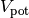) is given by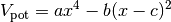where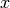is the position and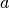,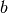and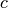are parameters for the potential.

Example Potential section for DoubleWell:
Potential
---------
class = DoubleWell
parameter a = 1.0
parameter b = 2.0
parameter c = 0.0


### Keywords for the DoubleWell potential¶

The following keywords can be specified for the DoubleWell potential:

Table 11 Keywords for the DoubleWell potential.
Keyword Description
class Selects the DoubleWell potential.
parameter Sets the parameters for the potential.

#### Keyword class¶

class = DoubleWell
This keyword selects the potential and should be set to: DoubleWell.

#### Keyword parameter¶

For the DoubleWell potential, three parameters can be specified:

parameter a = float

The a parameter in the potential energy function given above.

Default:
The default is: parameter a = 1.0
parameter b = float

The b parameter in the potential energy function given above.

Default:
The default is: parameter b = 1.0
parameter c = float

The c parameter in the potential energy function given above.

Default:
The default is: parameter c = 0.0

## The RectangularWell potential¶

This class defines a one-dimensional rectangular well potential. The potential energy is zero within the potential well and infinite outside. The well is defined with a left and right boundary.

Example Potential section for RectangularWell:
Potential
---------
class = RectangularWell
parameter left = -1.0
parameter right = 1.0


### Keywords for the RectangularWell potential¶

The following keywords can be specified for the RectangularWell potential:

Table 12 Keywords for the RectangularWell potential.
Keyword Description
class Selects the RectangularWell potential.
parameter Sets the parameters for the potential.

#### Keyword class¶

class = RectangularWell
This keyword selects the potential and should be set to: RectangularWell.

#### Keyword parameter¶

For the RectangularWell potential, two parameters can be set.

parameter left = float

The left border for the potential well function. The potential energy is 0 for positionssuch that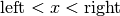.

Default:
The default parameter is: parameter left = 0.0
parameter right = float

The right border for the potential well function. The potential energy is 0 for positionssuch that.

Default:
The default parameter is: parameter right = 1.0

## The PairLennardJonesCutnp potential¶

This class implements as simple Lennard-Jones 6-12 potential which employs a simple cut-off and can be shifted. The potential energy () is defined in the usual way for an interacting pair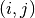of particles a distance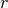apart,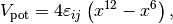where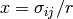and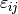and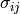are the potential parameters.

Example Potential section for PairLennardJonesCutnp:

Here is an example for defining parameters for a mixture of 3 particle types with geometric mixing.

Potential
---------
class = PairLennardJonesCutnp
shift = True
dim = 3
mixing = geometric
parameter 0 = {'sigma': 1.0, 'epsilon': 1.0, 'rcut': 2.5}
parameter 1 = {'sigma': 1.2, 'epsilon': 1.1, 'rcut': 2.5}
parameter 2 = {'sigma': 1.4, 'epsilon': 0.9, 'rcut': 2.5}


### Keywords for the PairLennardJonesCutnp potential¶

The following keywords can be specified for the PairLennardJonesCutnp potential:

Table 13 Keywords for the PairLennardJonesCutnp potential.
Keyword Description
class Selects the potential.
dim Set the number of dimensions to consider.
shift Determines if the potential should be shifted.
mixing Selects the mixing rules for generating parameters for cross interactions.
parameter Sets the parameters for the potential.

#### Keyword class¶

class = PairLennardJonesCutnp
This keyword selects the potential and should be set to: PairLennardJonesCutnp.

#### Keyword dim¶

dim = integer

Sets the dimensionality for the potential. Should be 1, 2, or 3 for 1D, 2D or 3D, respectively.

Default:
The default value is dim = 3.

#### Keyword shift¶

shift = boolean

Determines if the potential should be shifted or not. This should be either True (enables shifting) or False (disables shifting). If shifting is True, the following value will be added to the potential (for a givenpair):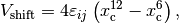where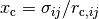and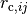is the cut-off.

Default:
The default value is shift = True.

#### Keyword mixing¶

mixing = string

Determines how mixing parameters for the potential should be determined. The supported mixing rules are:

• mixing = geometric:
•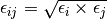•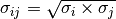•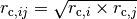• mixing = arithmetic:
••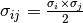•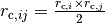• mixing = Sixthpower:
•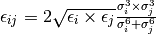•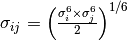•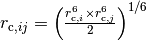Default
The default value is mixing = geometric.

#### Keyword parameter¶

The following parameters can be set for the PairLennardJonesCutnp potential:

parameter i = dictionary

The parameters for the PairLennardJonesCutnp potentials are given for each particle type on the form:

parameter i = {'sigma': 1.0, 'epsilon': 1.0, 'rcut': 2.5}


Where i is the particle type, identified as an integer. Here, sigma is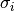, epsilon is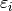and rcut is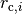.

Note that several parameters can be given in this way and that they will be combined according to the selected mixing rule.

## The DoubleWellWCA potential¶

This class defines a n-dimensional Double Well potential. The potential energy () for a pair of particles separated by a distanceis given by,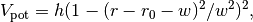where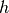gives the ‘height’ of the potential,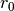the minimum and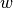the width.

Example Potential section for DoubleWellWCA:
Potential
---------
class = DoubleWellWCA
dim = 2
parameter rzero = 1.122462048309373
parameter height = 6.0
parameter width = 0.25
parameter types = [(1, 1)]


### Keywords for the DoubleWellWCA potential¶

The following keywords can be specified for the DoubleWellWCA potential:

Table 14 Keywords for the DoubleWellWCA potential
Keyword Description
class Selects the potential.
dim Sets the number of dimensions to consider.
parameter Sets the parameters for the potential.

#### Keyword class¶

class = DoubleWellWCA
Selects the potential, should be set to: DoubleWellWCA.

#### Keyword dim¶

dim = integer

Sets the dimensionality for the potential. Should be 1, 2, or 3 for 1D, 2D or 3D, respectively.

Default:
The default value is dim = 3.

#### Keyword parameter¶

The following parameters can be set for the DoubleWellWCA potential:

parameter zero = float

This is theparameter in the potential energy function given above.

Default
The default is rzero = 0
parameter height = float

This is theparameter in the potential energy function given above.

Default
The default is height = 0
parameter width = float

This is theparameter in the potential energy function given above.

Default
The default is width = 0
parameter types = list of tuples of integers

This parameter determines for which pair of particles the interaction should act on. If this is not set, it will turn on the interaction for all particles. For example, if types = [(1,2)] the interaction will be turned on between particles of type 1 and type 2 only. (See the particle section for a description about the particle type.) Note that several types can be specified at the same time: types = [(1, 2), (0, 1), (0, 2)].

Default
The default is activated for all pairs.

## User-defined potential functions¶

User-defined potential functions are selected as follows:

Example Potential section for a user-defined class:
Potential
---------
class = PotentialName
module = potential.py
keyword1 = value
parameter p = 123.


Here, the user can both define custom keywords and parameters. However, the class and the module must always be specified in this case.

### Keywords for user-defined potential functions¶

At least, the following keywords can be specified for user-defined potential functions:

Table 15 Keywords for user.defined potential functions.
Keyword Description
class Selects the Python class which implements the potential.
module Defines the file where the Python class with the potential can be found.

#### Keyword class¶

class = string
This keyword selects the potential function and it should be set to the class name of the custom potential function class defined in the given module.

#### Keyword module¶

module = string

This keyword specified the location of the file containing the user-defined class for the potential function. This file must be accessible by PyRETIS.

Default
Not any. This keyword must be specified.